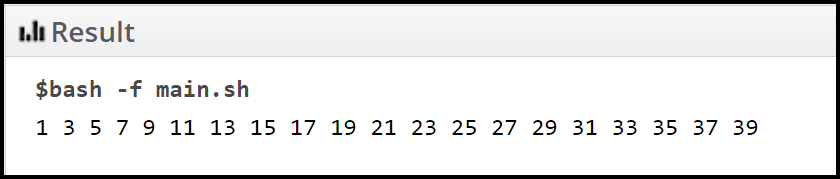# Shell Scripting – Command Substitution

• Last Updated : 27 Jan, 2022

A shell is an interface that helps users to connect with the system. Using a shell is equivalent to indirectly communicating with the operating system. In Linux distributed systems, each time we use the terminal, we connect with a shell. The job of a shell is to analyze Unix commands or instructions given by the user. This process involves taking commands from the user and converting them into a form that the kernel can easily understand. In simple words, it acts as a medium between the user and the kernel of the operating system. The kernel is the most crucial part of a computer’s operating system.

In order to understand command substitution, let us first discuss substitution in shell scripts. Substitution is a functionality by following which we can instruct the shell to substitute the actual value of an expression.

Example:

In the below program we have firstly created variable num and assigned it with the value 20 and then substituted the value of the num as 100 in the echo command.

```#!/bin/sh

num=100

echo -e "num: \$num"```

Output: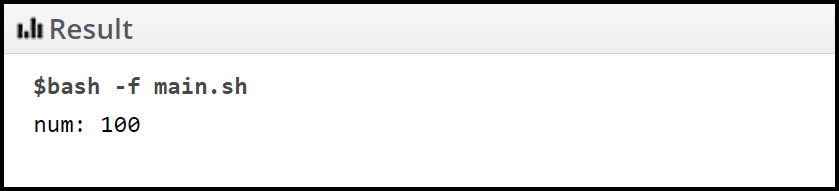This article focuses on the command substitution technique used in shell scripting.

There are some sequences of characters that don’t represent their true nature but they have special meaning to the operating system and these sequences are known as escape sequences. When they are used in a command, they are replaced by actual values.

## Command Substitution:

Command substitution is a mechanism that is followed by programmers in a shell script. In this mechanism, the output of a command replaces the command itself. Shell operates the expansion by executing a command and then replacing the command substitution with the standard output of the command. In simple words, the output of a UNIX command is bundled and then used as a command.

To understand it in a better way, let us consider an example. The seq command in Linux is used to print numbers from START to END in steps of INCREMENT.

Syntax:

`seq START INCREMENT END  `

Return type:

Prints numbers from START to END each in the new line by the difference of INCREMENT.

Example:

In the below script we are printing numbers from 2 to 40 with a difference of 2. In other words, we are printing even numbers up to 40.

```#!/bin/sh

seq 2 2 40```

Output: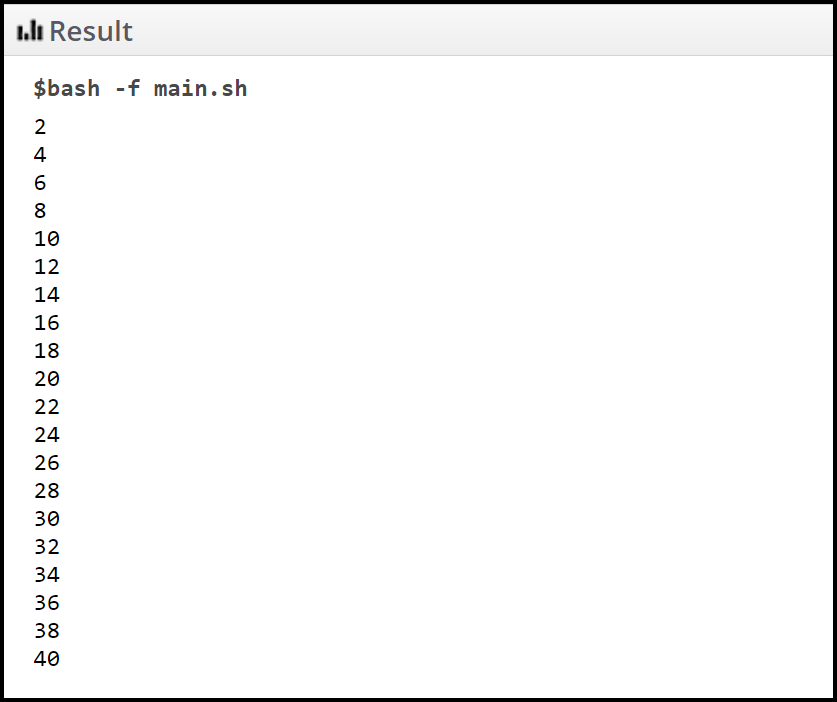We can use the output of the above command as a new command. Consider the below script,

Example:

```#!/bin/sh

echo \$(seq 2 2 40)```

Output: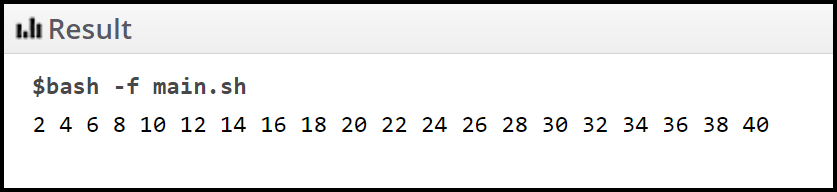## Variables and command expansion:

During the process of command substitution, the output of the command can be assigned to a variable, just like any other value.

Example:

In the below script we have assigned the result of the echo command to both the strings in variables, “variable1” and “variable2” respectively. Then we have used these variables in the echo command.

```#!/bin/sh

variable1=\$(echo 'Full form of gfg is' )

variable2=\$(echo 'GeekforGeeks')

echo "\$variable1 : \$variable2"```

Output: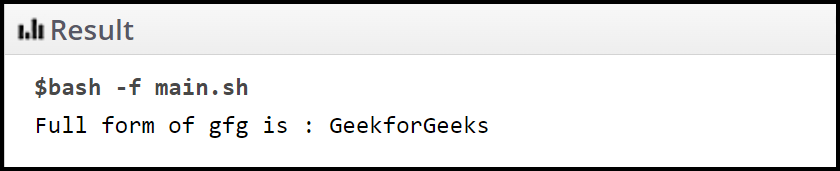## Loss of newlines in command substitution:

In the command substitution mechanism, If the output of a command that is being substituted contains any trailing newlines, then in that case the trailing newlines are deleted after the substitution. Note that embedded newlines are not deleted, but they might be removed during word splitting.

Example:

In this script, we are using the seq command. Now seq command prints numbers to the console and appends a newline character after each number is printed to the console.

```#!/bin/sh

seq 1 2 19```

Output: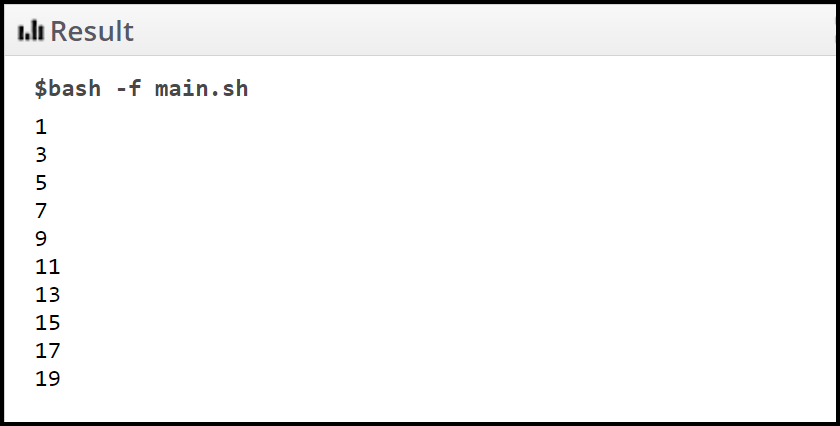Example:

In this script, we have used the result of the above command and substituted it in the command. As you can see in the output, numbers from 1 to 39 with the difference of 2 are printed to console. But this time they all are printed on the same line that is without any newline character.

```#!/bin/sh